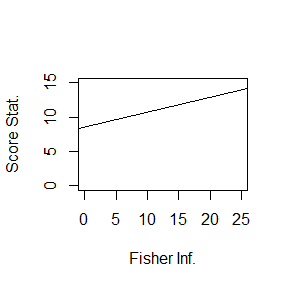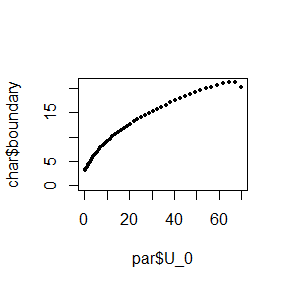Design and Analysis of Clinical Trials with Fully Flexible Adaptive Sample Size Determination

2018-09-20

In this vignettes, an application of a locally and a globally efficient adaptive sample determination to a confirmatory randomized clinical trial is illustrated.

Clinical Trial Example

This trial evaluated whether oral adjuvant chemotherapy with tegaful and uracil (UFT) and leucovorin (LV) reduces the recurrence after resection of liver metastasis from colorectal carcinoma as compared with no adjuvant therapy in Japan (UFT/LV trial) (Hasegawa et al. PLoS One 2016;11:e0162400.). The null hypothesis $$log(HR) = 0$$ was tested with the one-sided significance level of 0.025. The minimum of clinically important effect size was hypothesized as HR = 0.65. The test statistic was a stratified log-rank score. Suppose that four interim analyses and one final analysis were planned to be performed but when to perform was not fixed in advance.

The result of the interim analyses were as follows. * Fisher information at analyses: (5.67, 9.18, 14.71, 20.02) * Score statistic = (3.40, 4.35, 7.75, 11.11)

Locally efficient adaptive design

The initial working test (SPRT) is prepared as a basis of conditional error function. Its stopping boundary is $$-\log(\alpha) / \rho + 1 / 2 \rho t$$, where the significance level $$\alpha = 0.025$$ and the minimum of clinically important effect size $$\rho = -log(0.65)$$ will be substituted and $$t$$ is the Fisher information. This stopping boundary is depicted below.The four interim analyses can be performed by the function adaptive_analysis_norm_local. Designating FALSE to the argument final_analysis indicates that the latest analysis is not the final, i.e., the overall significance level must not be exhausted at this time.

The result is summarized as follows:

At the forth (final) interim analysis, the null hypothesis is not rejected. Then, the maximum sample size (here, the maximum Fisher information level) is calculated. The alternative hypothesis for which an adequate level of power will be ensured can be determined arbitrarily referring to all available data including the interim results but not correlates of future data. Here, the maximum likelihood estimate $$11.11 / 20.02$$ at the forth interim analysis is chosen as the alternative hypothesis. The maximum information level to obtaine the marginal power of 0.75 can be calculated by the function sample_size_norm_local.

Finally, suppose that the final analysis is performed at $$t = 24.44$$. The same function used at interim analyses, adaptive_analysis_norm_local, can be used with setting final_analysis = TRUE.

Again, the result is summarized as:

As indicated at the final row, the null hypothesis is rejected.

Globally efficient adaptive design

Globally efficient adaptive design can be performed in a similar way by using the functions for globally efficient functions.

The initial working test, a group sequential design with 50 analyses, is prepared as a basis of conditional error function. Its stopping boundary can be constructed by the function work_test_norm_global.

Here, cost_type_1_err = 0 means the value of loss caused by erroneous rejection of the null hypothesis is calculated to make the constructed working test have exactly the type I error probability of $$\alpha$$. The default value of cost_type_1_err is 0 and thus can be omitted. The boundary of the working test just constructed is displayed by the next code.The four interim analyses can be performed by the function adaptive_analysis_norm_global. Designating FALSE to the argument final_analysis indicates that the latest analysis is not the final, i.e., the overall significance level must not be exhausted at this time.

The result is:

At the forth (final) interim analysis, the null hypothesis is not rejected. Then, the maximum Fisher information level is calculated. The maximum likelihood estimate $$11.11 / 20.02$$ at the forth interim analysis is chosen as the alternative hypothesis, though this is not compelling. The maximum information level to obtaine the marginal power of $$0.75$$ can be calculated by the function sample_size_norm_global.

Finally, suppose that the final analysis is performed at $$t = 25.88$$. The same function used at interim analyses can be used, with setting final_analysis = TRUE.

As indicated by the final row, the null hypothesis is rejected.

Note that, if estimate = TRUE, additionally exact P-value, median unbiased estimate, and confidence limits can be calculated. These results will be extracted by: